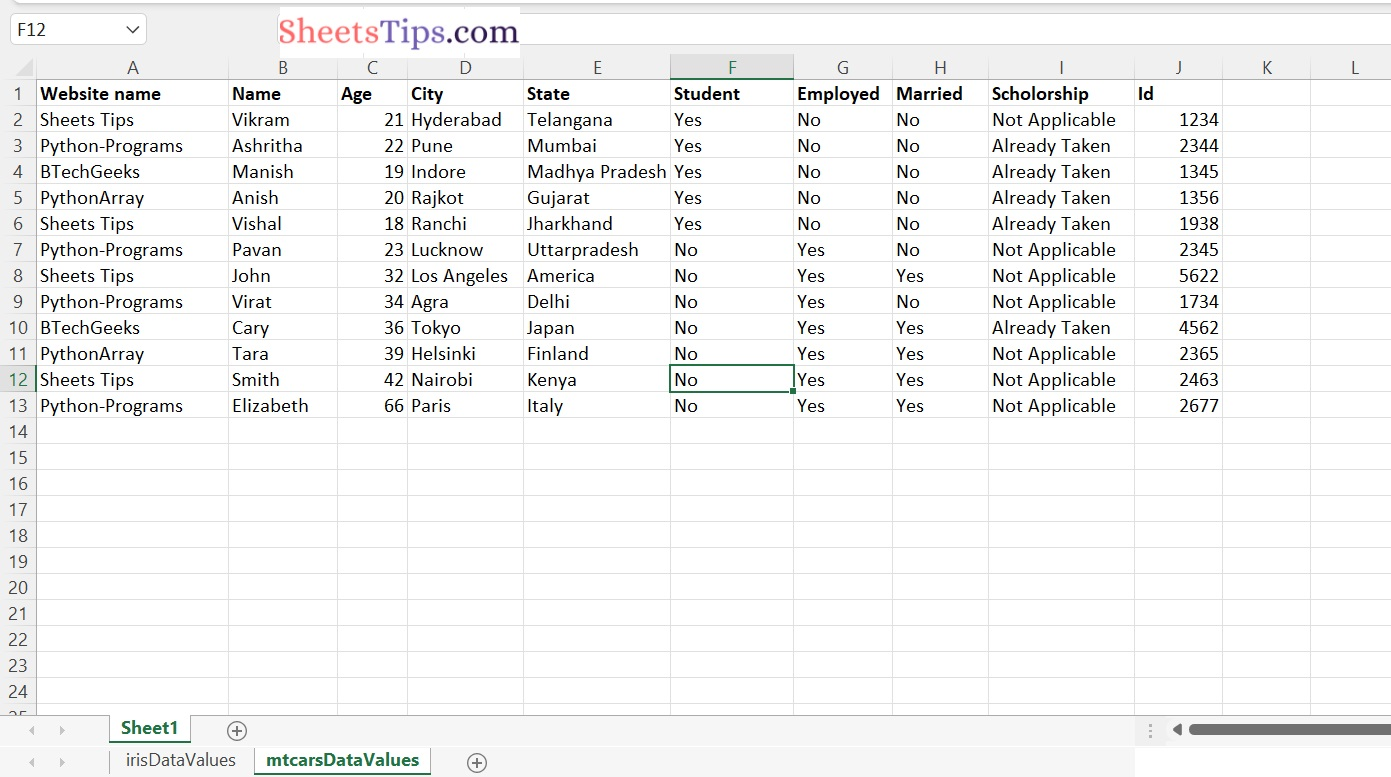# How to Count Number of Rows and Columns in an Excel file in Python?

In this article, let us look at how to count the number of rows and columns in an excel file using python.

For this purpose, we make use of the xlrd library in python here.

Let us use the `sampleExcelFile.xlsx` file here which has the following data:

sampleExcelFile:#### Python xlrd Module:

The xlrd module can be used to get data from a spreadsheet. It can be used to read, write, or modify data. In addition, the user may have to navigate through several sheets and get data based on some criteria, or edit some rows and columns, among other things.

To extract data from a spreadsheet, use the xlrd module.

The Python xlrd library, which is available on Pypi, is a library that allows users to read Excel files with the extensions “.xls” or “.xlsx.” Before you move into the code, be sure to install it.

NOTE:

``` Support for reading xlsx sheets has been explicitly removed from xlrd module.
```

Installation:

Type the below command to install the xlrd module:

```pip install xlrd
```

Functions Used:

• open_workbook(): It is used to open a workbook.
• sheet_by_index(): This function is used to access/open the sheet with a specific index number.
• nrows: It is used to give the total number of rows.
• ncols: It is used to give the total number of rows.

## Python Program to Count Number of Rows and Columns in an Excel file in Python

Step#1: Importing the module

Import xlrd module using the import keyword to work with the excel file.

```# Import xlrd module using the import keyword
import xlrd```

Step#2: Input the excel File

Here we input an excel file on to count the numbers of rows and columns in an excel file by giving the path of the excel file.

```# Give the path of the excel file to be read as
# static input and store it in a variable
excelFilePath="sampleExcelFile.xlsx"```

Step#3: Creating a workbook and a worksheet

Here we create a workbook for the given excel file using the open_workbook() function and also access the required worksheet using the sheet_by_index() method.

```# Pass the above file path as an argument to the open_workbook() function
# of the xlrd module to create/open a workbook.
workbook=xlrd.open_workbook(excelFilePath)
# Open a first worksheet in the above workbook using the sheet_by_index() function
# by passing the index value 0 as argument to it.
# you can open any other sheets just by changing the index number(starts from 0)
worksheet=workbook.sheet_by_index(0)```

Step#4: Finding the number of rows and columns in an excel file

Now, let us calculate the count of the numbers of rows and columns in an excel file using the below part of the code.

```# Initializing a cell from the excel file referenced via the cell location
# Here we passed row number as 0 and column number as 0
# Because we want to get all the number of rows and columns from the start of the excel file
worksheet.cell_value(0,0)
# Print the number of rows in a worksheet using the nrows attribute
print("The number of rows in a worksheet = ", worksheet.nrows)
# Print the number of columns in a worksheet using the ncols attribute
print("The number of columns in a worksheet = ", worksheet.ncols)```

#### Complete Code

Here is the complete code for you.

Approach:

• Import xlrd module using the import keyword
• Give the path of the excel file to be read as static input and store it in a variable
• Pass the above file path as an argument to the open_workbook() function of the xlrd module to create/open a workbook.
• Open the first worksheet in the above workbook using the sheet_by_index() function
by passing the index value 0 as argument to it.
• You can open any other sheets just by changing the index number(starts from 0)
• Initializing a cell from the excel file referenced via the cell location
• Here we passed row number as 0 and column number as 0.
• Because we want to get all the number of rows and columns from the start of the excel file
• Print the number of rows in a worksheet using the nrows attribute
• Print the number of columns in a worksheet using the ncols attribute.
• The Exit of the Program.

Below is the implementation:

```# Import xlrd module using the import keyword
import xlrd
# Give the path of the excel file to be read as
# static input and store it in a variable
excelFilePath="sampleExcelFile.xlsx"
# Pass the above file path as an argument to the open_workbook() function
# of the xlrd module to create/open a workbook.
workbook=xlrd.open_workbook(excelFilePath)
# Open a first worksheet in the above workbook using the sheet_by_index() function
# by passing the index value 0 as argument to it.
# you can open any other sheets just by changing the index number(starts from 0)
worksheet=workbook.sheet_by_index(0)
# Initializing a cell from the excel file referenced via the cell location
# Here we passed row number as 0 and column number as 0
# Because we want to get all the number of rows and columns from the start of the excel file
worksheet.cell_value(0,0)
# Print the number of rows in a worksheet using the nrows attribute
print("The number of rows in a worksheet = ", worksheet.nrows)
# Print the number of columns in a worksheet using the ncols attribute
print("The number of columns in a worksheet = ", worksheet.ncols)```

Output:

```The number of rows in a worksheet = 13
The number of columns in a worksheet = 10